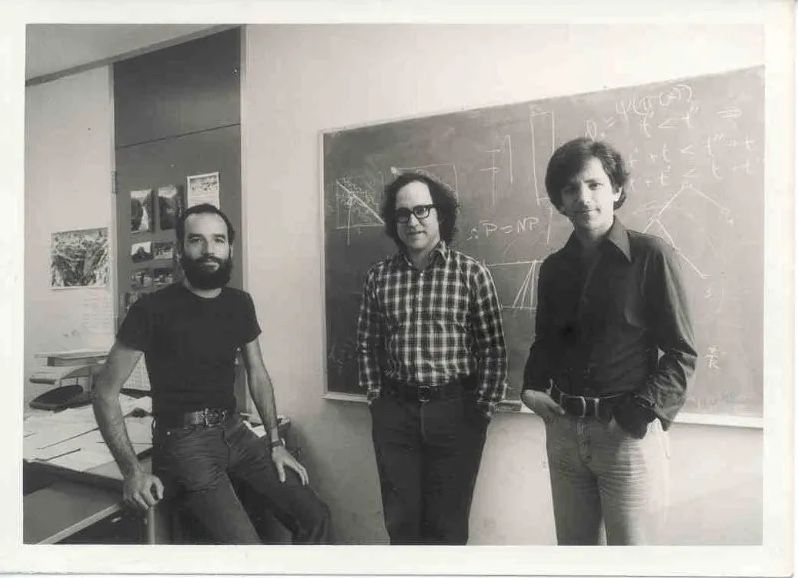RSA is an asymmetric key encryption algorithm and was first published in 1977.RSA works on the concept of modular arithmetic.

In RSA, we have a public and a private key. We use the private key to decrypt and the public key to encrypt.

This means that the private key should not be shared. But the public key can be shared with anyone.

Private Key = Key Public Key = Lock

There are 5 steps in RSA.

1. Take two prime numbers p and q. (Eg: p=2, q=7)
2. Calculate N . N is the product of p and q. (N = p*q = 2*7 = 14)
3. Calculate Phi(N) .
4. This is the number of co-primes less than N. (Eg. 1, 2, 5, 7 and 11 are co-primes to 14; therefore Phi(n) is the count = 6)
5. This can also be calculated with a formula (p-1)(q-1)
6. Eg; Phi(N) = (2-1)(7-1) = 6
7. Create the public key (e) such that
8. 1 < e < Phi(N)
9. E should be co-prime with Phi(N)
10. Eg. e = 5
11. Find the private key (d) such that
12. (e*d) mod Phi(n) = 1
13. Eg: (5*d) mod (14) = 1
14. When you calculate this you’ll see a pattern.
• 5*1 = 5; mod 6 = 5
• 5*2 = 10; mod 6 = 4
• 15 mod 6 = 3
• 25 mod 6 = 1
• 30 mod 6 = 0 This pattern is expected since (5*6)%6 will be 0.

Let’s pick a large d .

D = 11

Not to encrypt you’ll do the following,

(value^e) mod N


To decrypt, you’ll do the following,

(encryptedValue ^d) mod N


## Resources

Eddie Woo: The RSA Encryption Algorithm (1 of 2: Computing an Example)

The RSA Encryption Algorithm (2 of 2: Generating the Keys)

RSA Calculator